# How to calculate compound interest in Google SheetsThe system of compound interest is used by banks when issuing deposits, and is also used in various types of investment activities. At the same time, it is very difficult to assess the full power and effectiveness of such a tool, especially if you take into account the fact that possible additions are made every period. If you need to calculate a complex percentage yourself, you can make a simple table in Google Sheets and enter different values ​​to calculate the probable return.

## Calculation of compound interest with attachments in Google Sheets

Let’s start with a more complicated option, when in addition to accruing interest on the initial deposit, the client or investor deposits a certain amount into the compound interest account every month. In this case, it will also be included in the compound interest itself and will be counted together with the bonuses already accrued for the period. Such calculations will require a simple table with several columns.

### Preparatory actions

I created a sample version of what the table looks like with similar calculations. You can completely copy it or customize it for yourself. Let’s briefly explain the purpose of each column.

1. I created a column “Moon” only for convenience, since I additionally use a line with names. If you want, you can count the months yourself or start from the numbering on the sheet of the spreadsheet itself.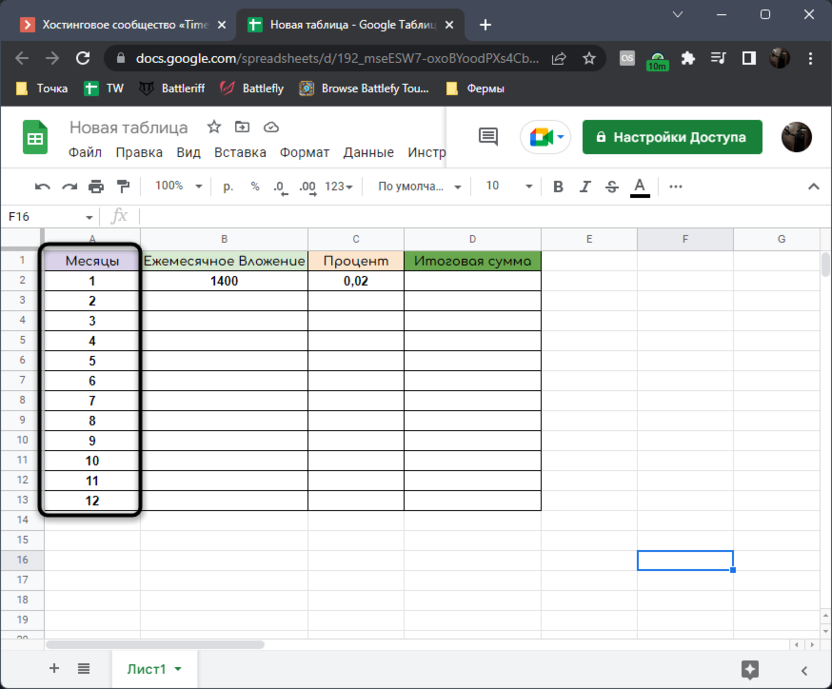2. Next comes the column with attachments. In this case, it is possible to write down not a fixed amount, but any number every month, making the contribution more flexible.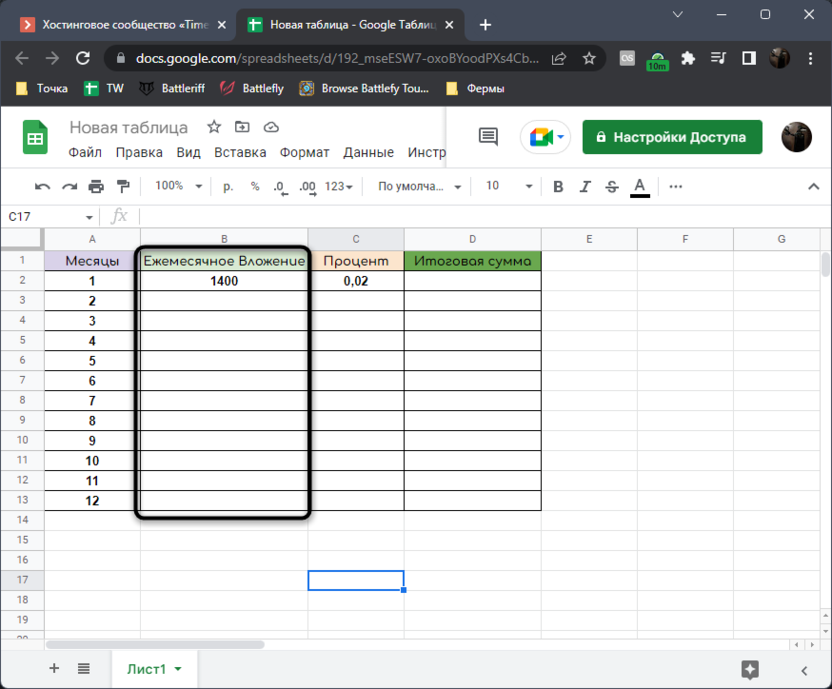3. The percentage can also be flexible if needed. A corresponding column is allocated for it. For convenience, I indicate the percentage as a decimal fraction, i.e 2% – this 0.02. If you want, you can just use any number with a sign %Google Sheets directly recognizes this type of cells.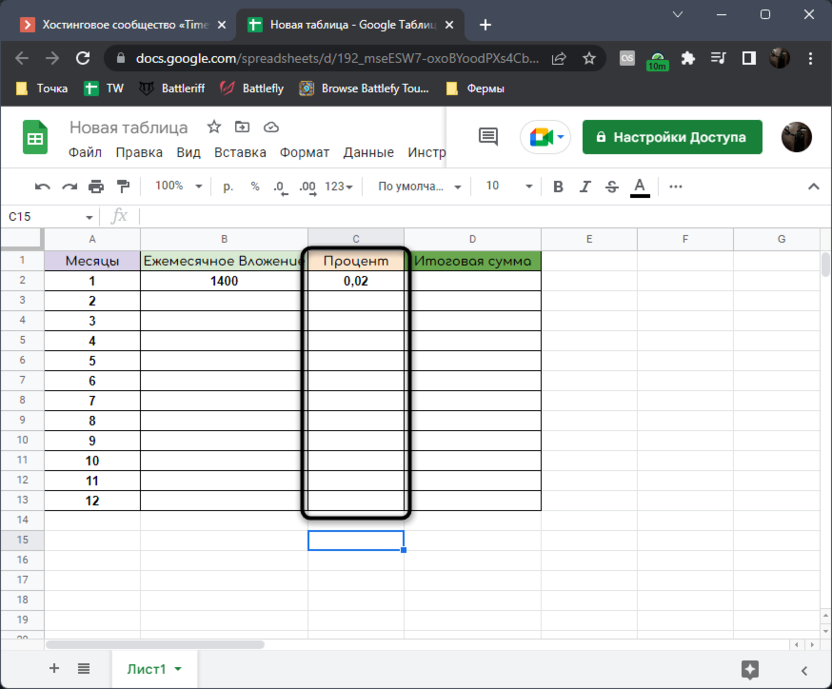4. In the column “Final amount” the reward that the depositor receives at the end of each period will be shown. It will still be useful for the subsequent formula, since the compound percentage of the next one will be calculated from the total amount of the last month or any other period.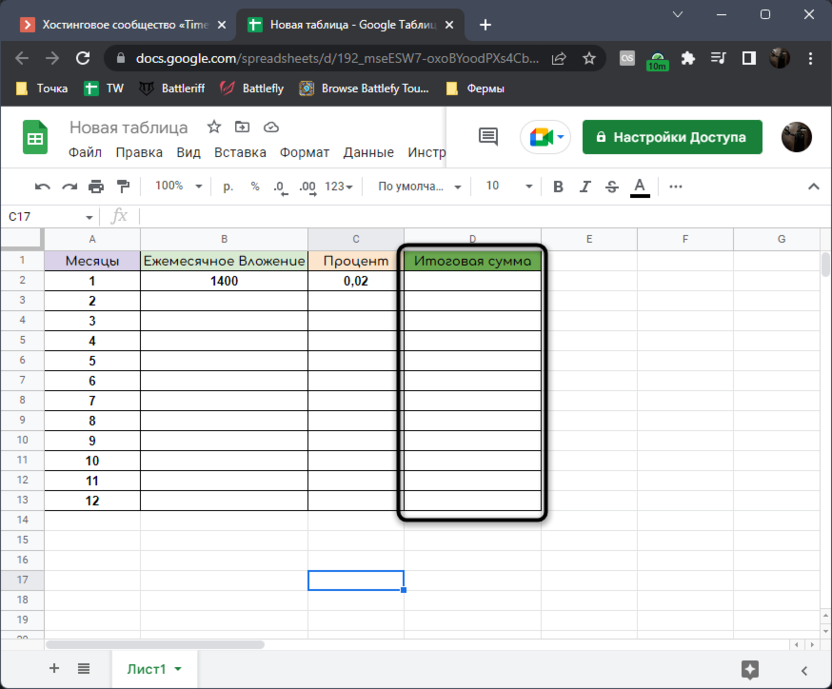These are fairly standard inputs that require compound interest calculations with monthly, weekly, or annual top-up on the available balance. Next, when the table is already built, you can start calculations.

### Creation of formulas

You only need to use two simple formulas. The first will help determine the amount of accruals for the first period, and the second will calculate the amounts of the rest of all periods, collecting information from previous cells. I will clearly demonstrate everything in the following instructions.

1. In the cell of the total amount for the first month, enter the formula =B2*C2. This will allow us to calculate the percentage of the initial deposit amount. In my case it works 1400 * 0.02, and yours will have completely different numbers. Accordingly, they must already be written in their cells to be referenced.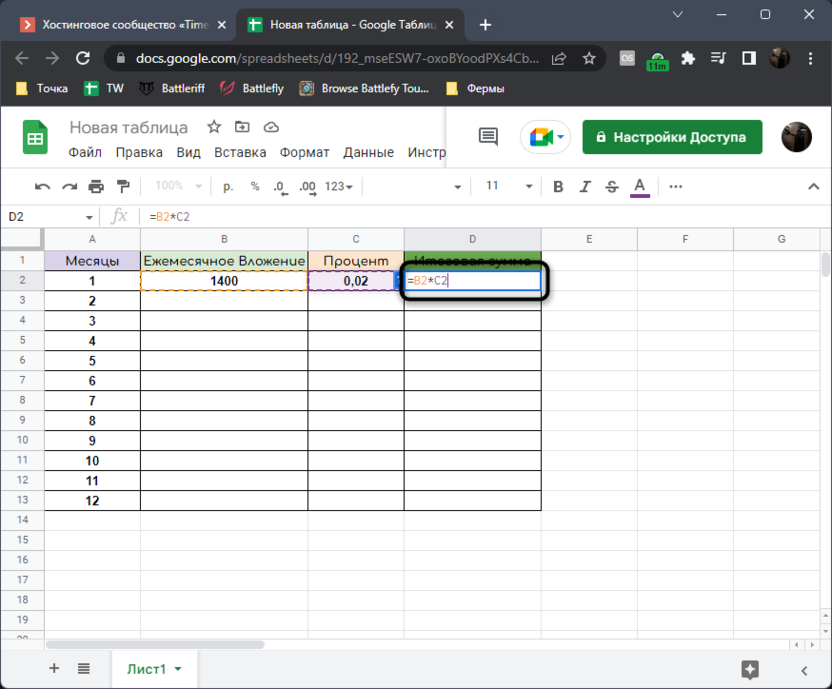2. Next, we simply add to this formula +B2that is, we add the initial amount of the deposit to the accrued interest.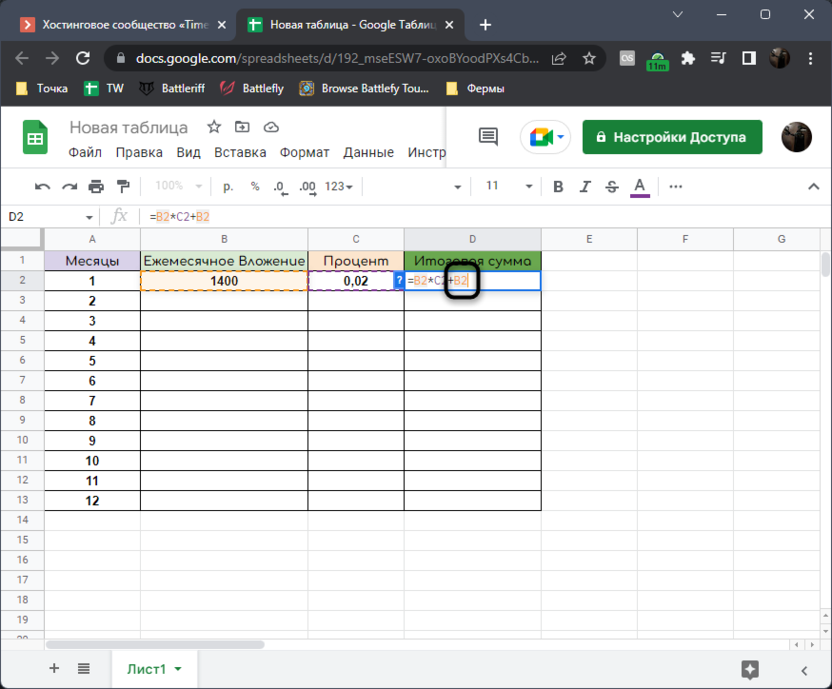3. Click Enter to apply the formula and see the result. In my case 2% from 1400 it turned out 28that is, at the end of the first period, there is already on the balance sheet 1428.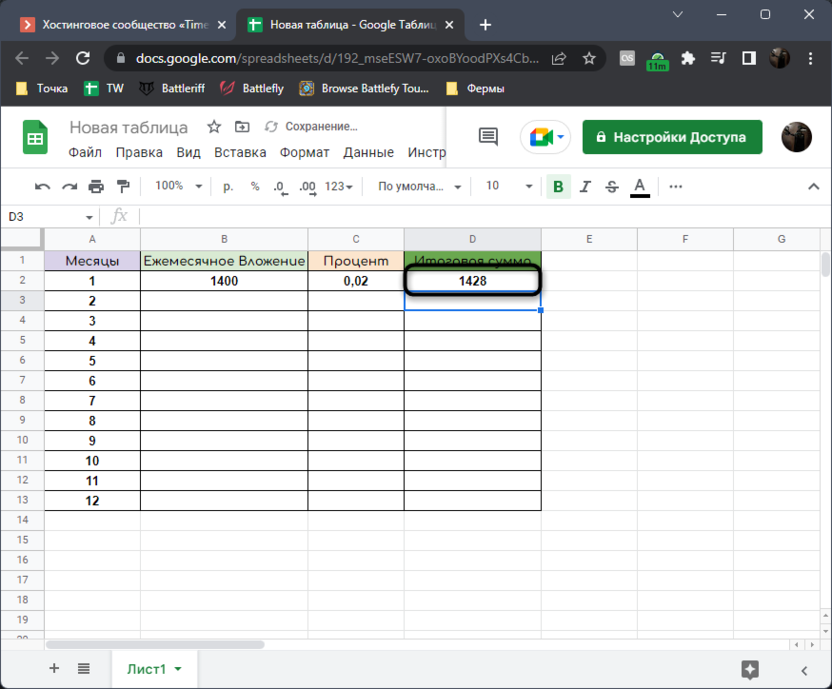4. Now you need to fill in a slightly more complicated formula. To do this, declare it in the cell of the next period with the final amount by writing =(D2+B3). This will calculate the amount for the previous period with your addition in it.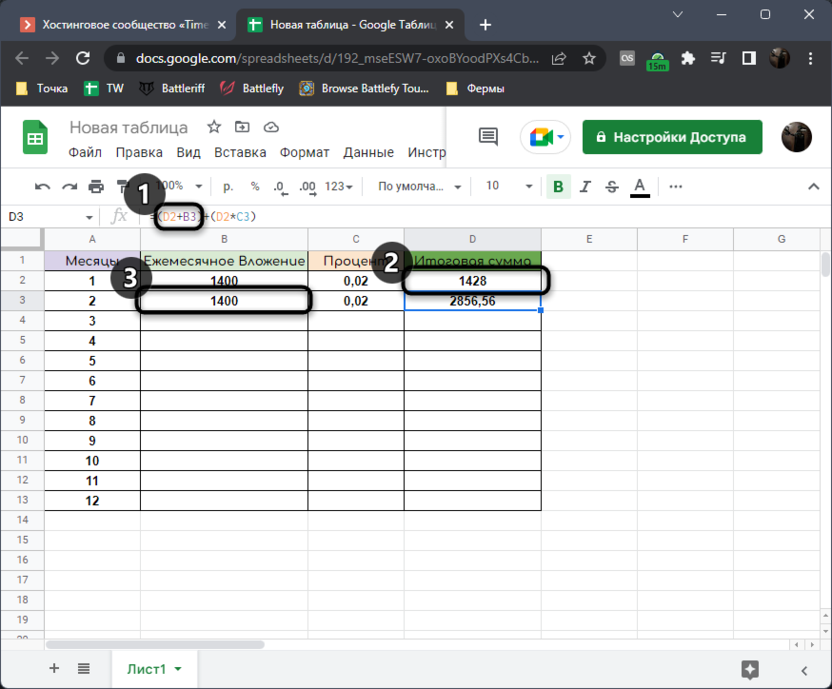5. Now, based on the available funds in the deposit account, you will need to find out what percentage will be charged next month. For this we add +(D2*C3). It turns out that we multiply the final amount of the past period by a fixed or floating interest, obtaining the same profit from compound interest.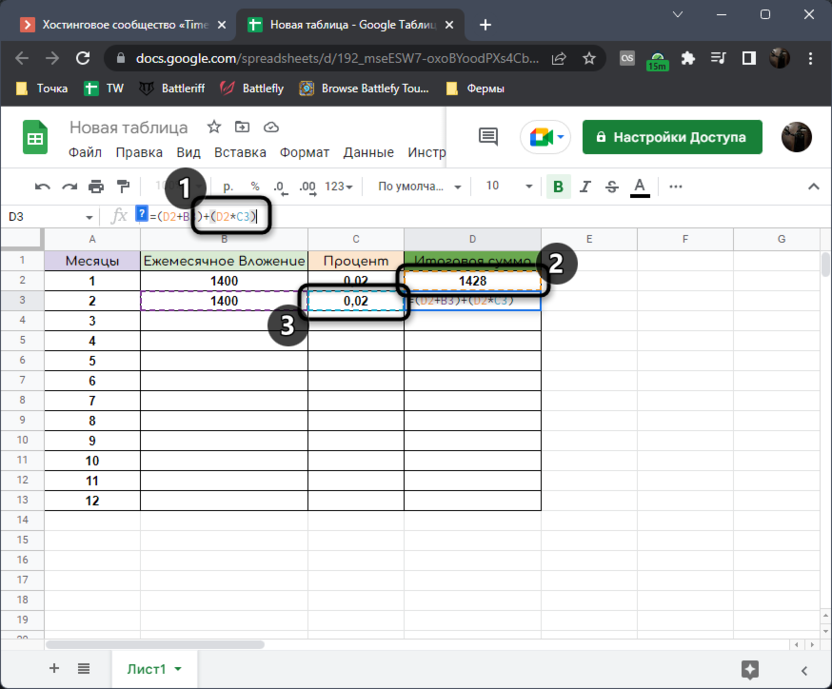6. Click Enter and make sure the formula worked correctly.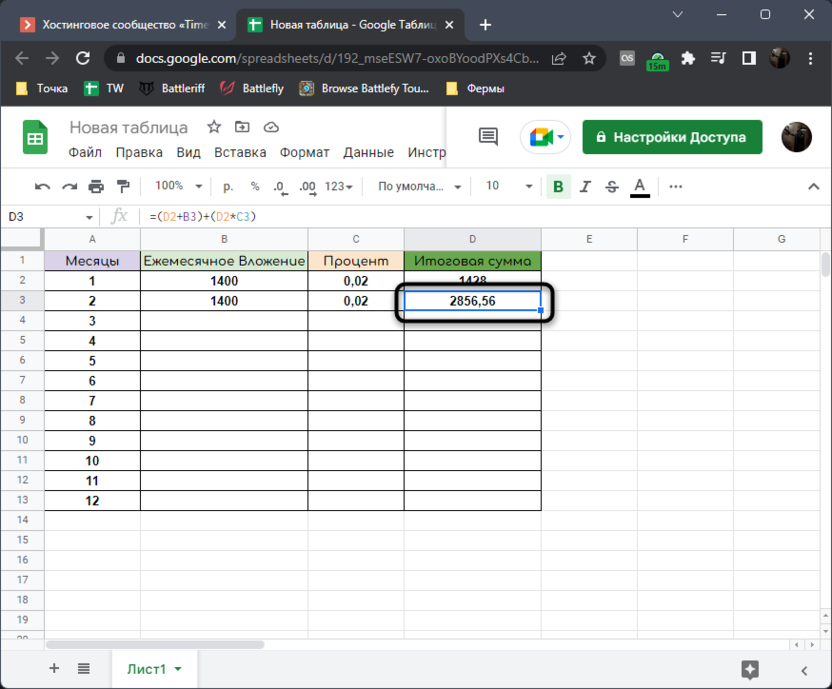The whole formula completely looks like =(D2+B3)+(D2*C3). Accordingly, if you have data recorded in other cells, their letter references will need to be changed. In the first quotes, we add your top-up amount to the amount that is already in the deposit account. Next, we add the percentage calculated based on the sum of the second period. I hope I have written everything as simply and clearly as possible. Now let’s move on to expanding our table.

1. If your percentage is floating, enter each value for each period cell separately. If it is fixed, you can simply stretch the already entered value down by pinching the cell by the lower right corner. This also applies to permanent refills, where you enter the same value or different values, depending on your goals and capabilities.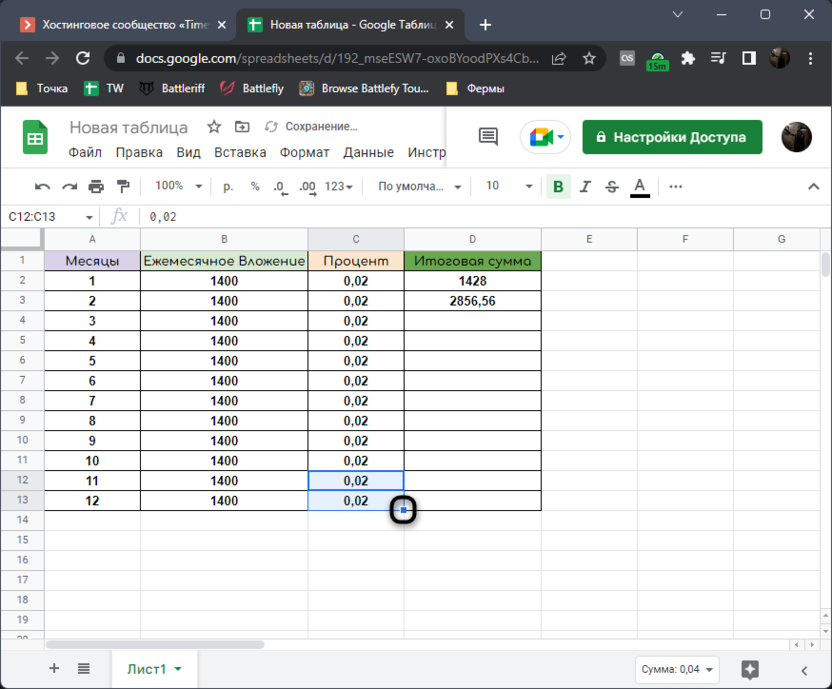2. The same should be done with the cell of the final amount, stretching the formula to the end of the entire accrual period. You will see how the calculations took place automatically, and you no longer need to do anything at all, because you already know the final amount that you will receive from this method of additions with compound interest.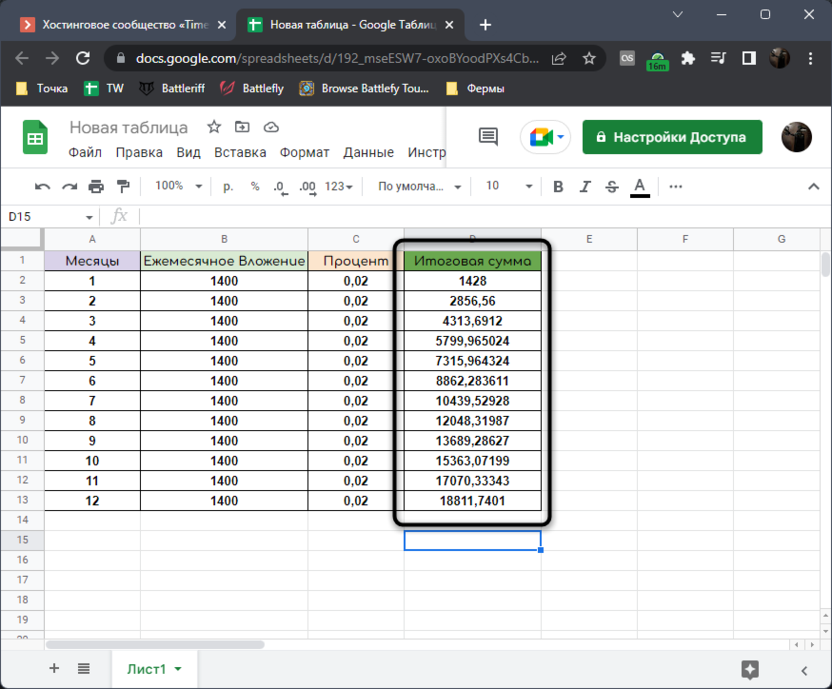3. If you need to remove extra decimal places, click the button on the top panel, which shows an arrow with a zero.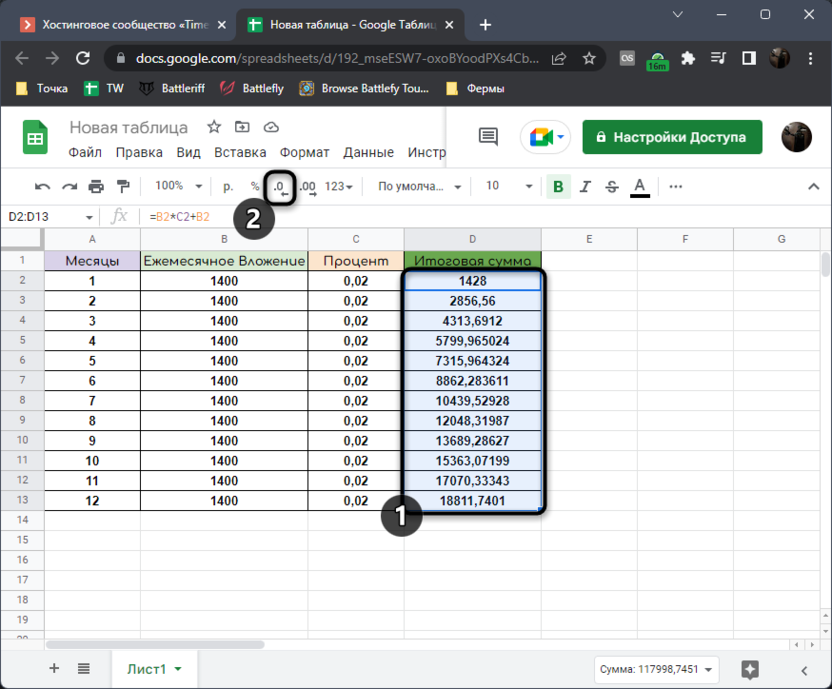The community is now in Telegram

Subscribe and stay up to date with the latest IT news

## Calculation of compound interest without applications in Google Sheets

Now let’s consider a simpler option. In this case, the compound interest will be calculated without regular replenishment of the initial payment. Let’s say you put 5,000 rubles in an account with a monthly income of 5%. At the same time, this 5% is immediately added to the initial deposit, and the interest in the next period already takes into account the new amount, and so on until you withdraw money from this account or the investment mechanism is closed. Let’s create a table for this situation.

### Preparatory actions

Again, I will briefly dwell on which table I will use for the following formulas. You can make it the same or optimize it for yourself.

1. I again indicate the months, which are periods, on my own, since the calculation does not start from the beginning of the letter on the table.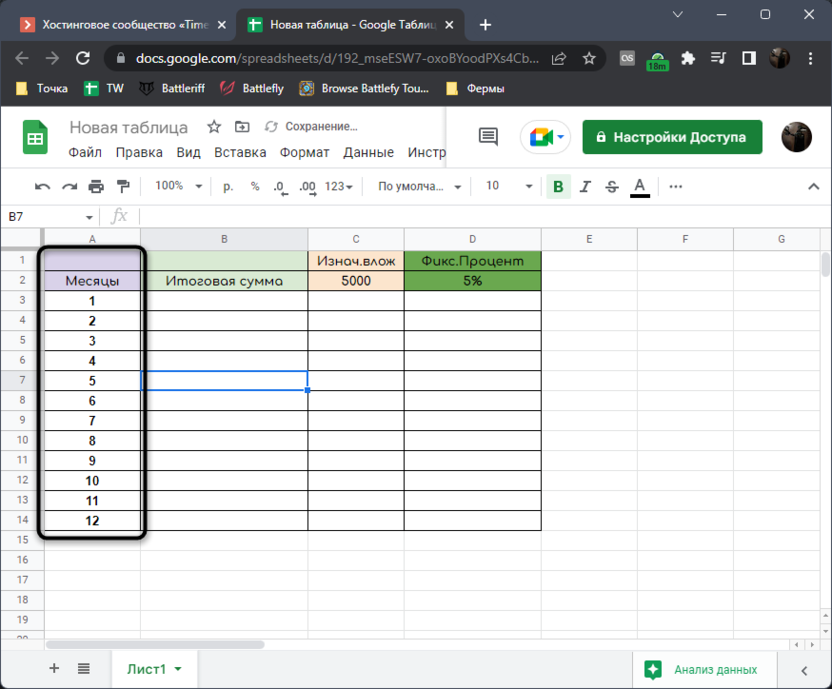2. I am adding a column “Final amount”always know how much money will be in the account at the end of each period.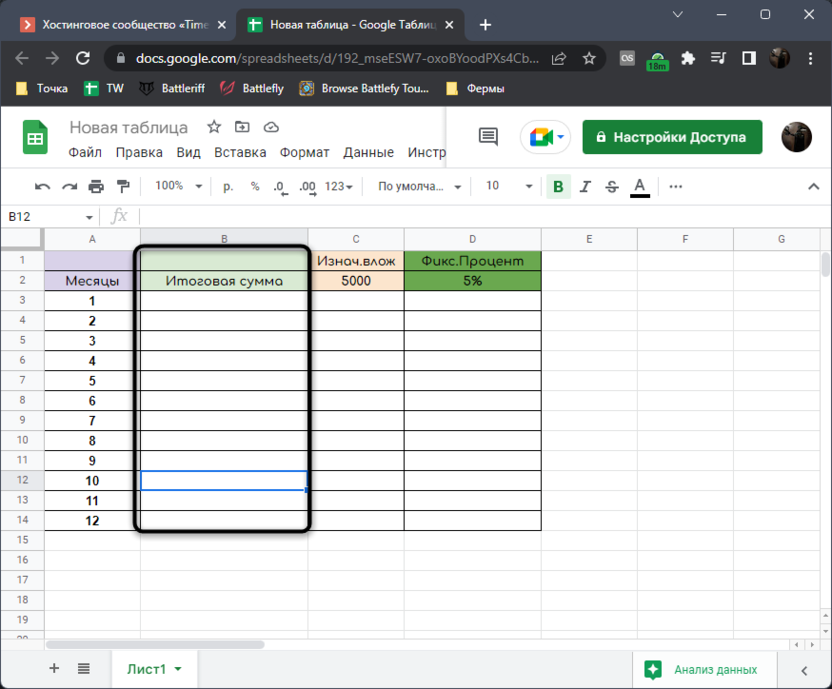3. To the right of clarity, I indicate the amount of initial investments, as well as a fixed percentage that will be added monthly to the amount on the balance sheet. A number with a percentage is required because we will use it for our formula later.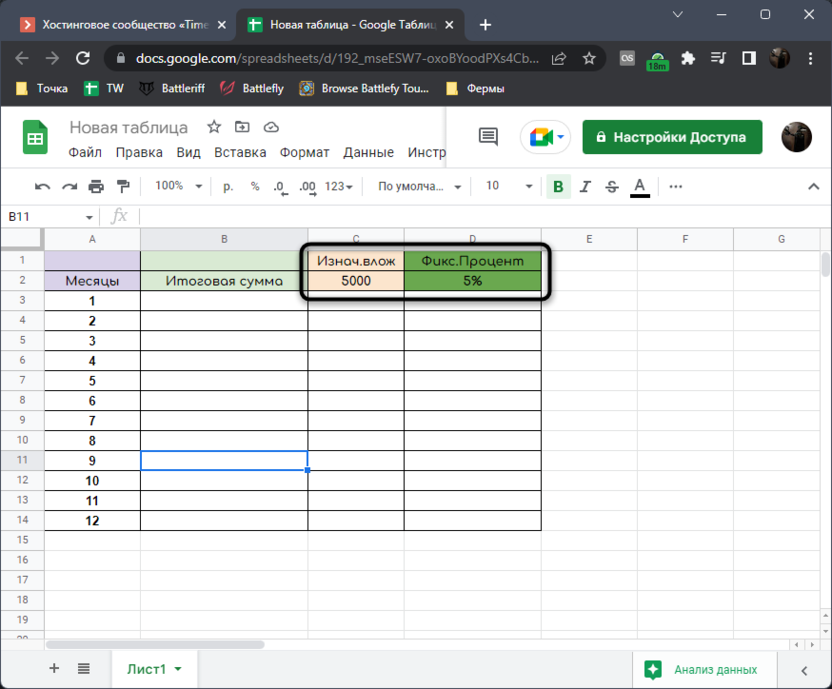Now that the table itself is there, you can start filling it in, which will be a difficult task.

## Creating a formula

In this case, there is less initial data, so the formula will not be so complicated. First, you need to calculate the result for the first period, and then extend the action of the formula to the entire remaining table.

1. In the first cell where the amount will be shown, enter the initial investment and multiply it by the indicated percentage.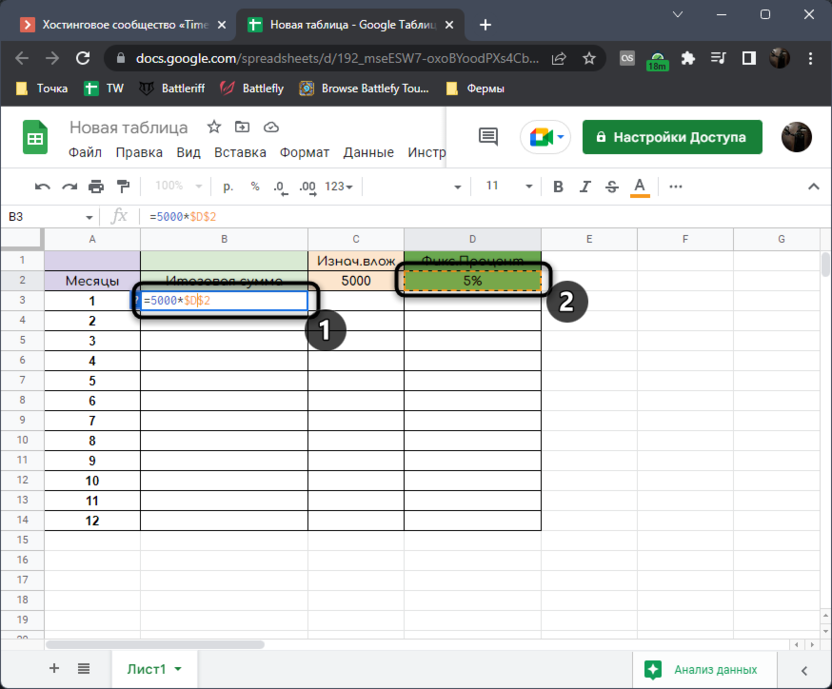2. Click Enterto know the result.3. Go back to editing the formula and add the initial investment amount to it to get the result of savings for the month.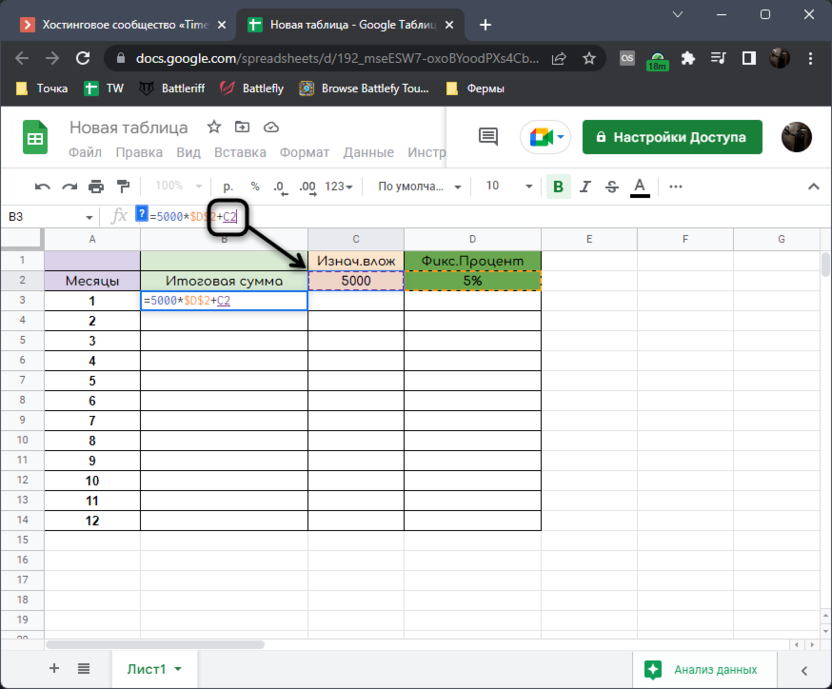4. The formula will come out =5000*\$D\$2+C2. First, the percentage is determined, and the deposit amount is added to it. You can either refer to the cells or enter the values ​​manually as numbers, if that’s more convenient.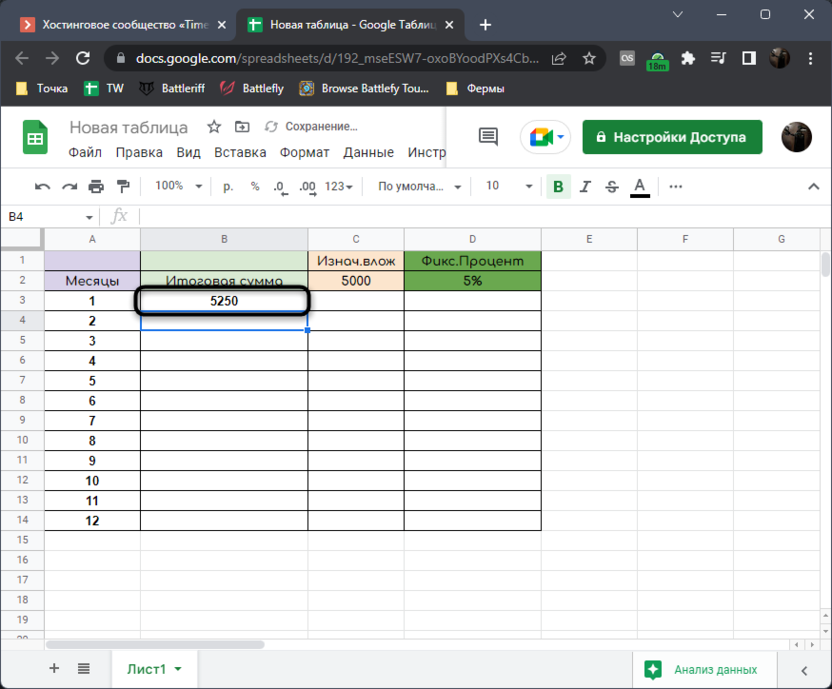5. Now you need to independently create a formula for the next period in order to further stretch it and get an accurate result. For this use = B3 * \$ D \$ 2 + B3. Here we refer to the amount received for the previous period, calculate the percentage and add the amount to get the total for this month. The cell reference with the percentage is a constant (\$D\$2). This is necessary so that when the formula is further stretched, it does not shift.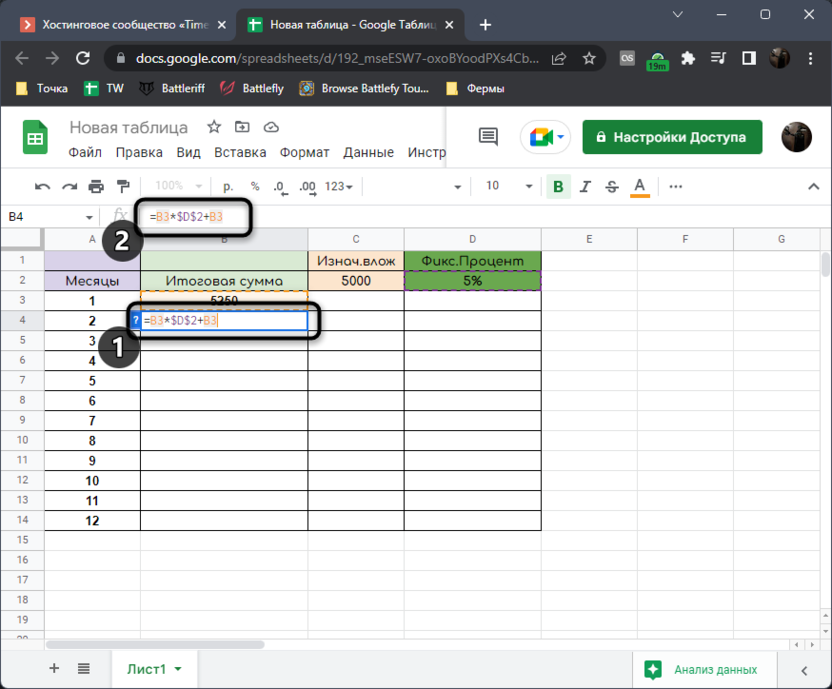6. Click Enter and check if the formula considers compound interest.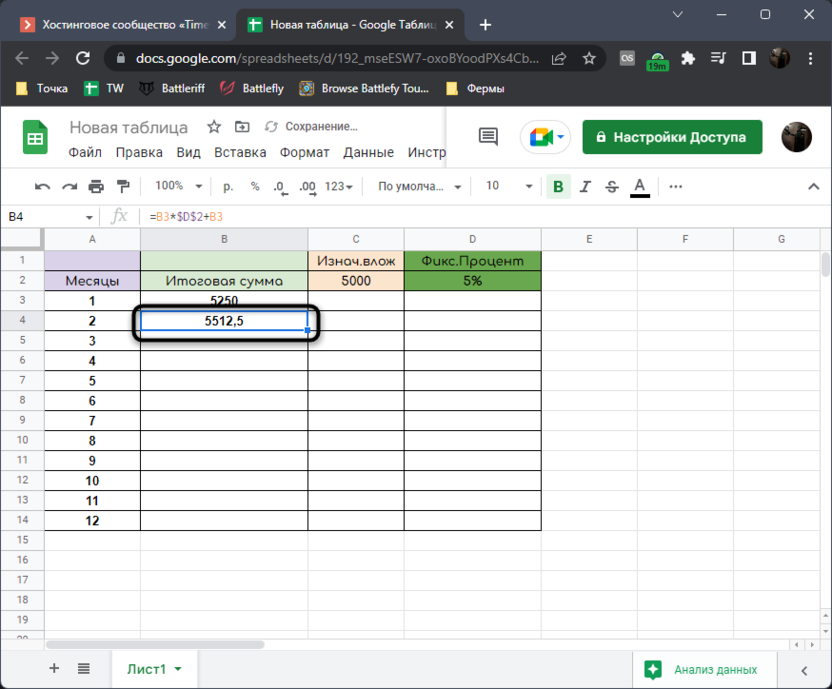7. Left-click this cell by the lower right corner and drag down.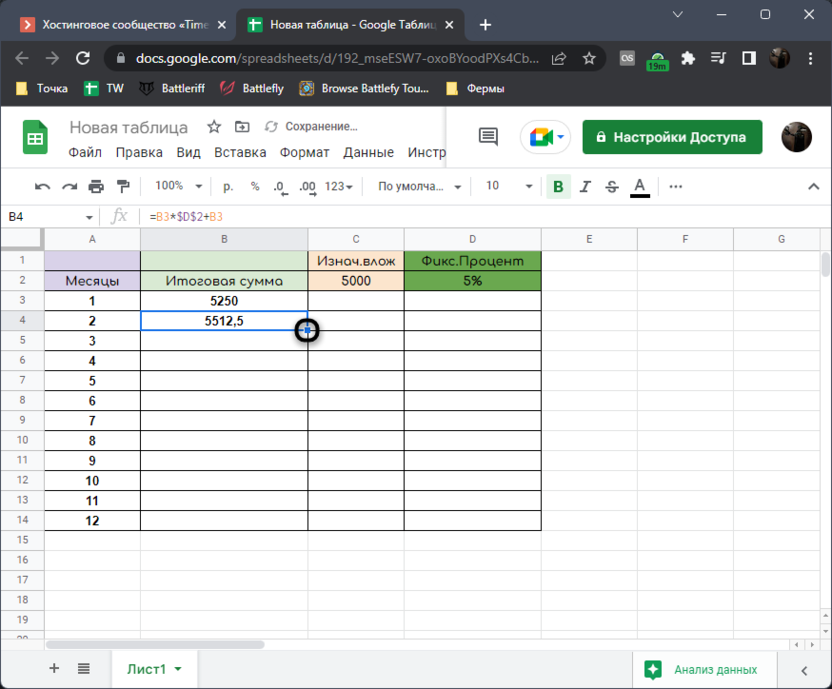8. After scaling the formula, you will get the compound interest result for all periods and you will be able to understand how profitable this type of investment is.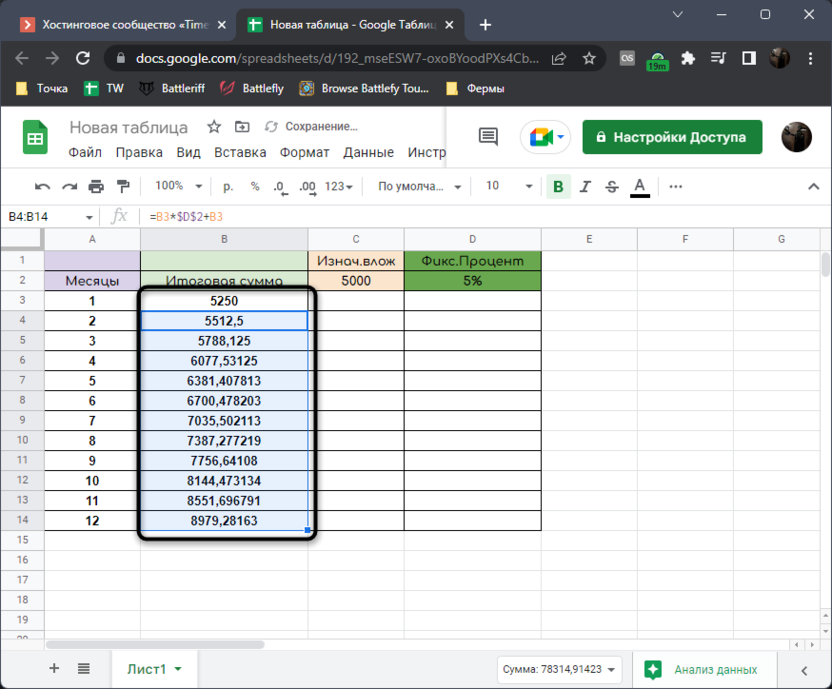Here are two simple tables we got. I hope that with the help of the instructions, you have improved your skills in working with electronic documents created in Google Sheets, and now you know how to calculate compound interest for calculating profitability.